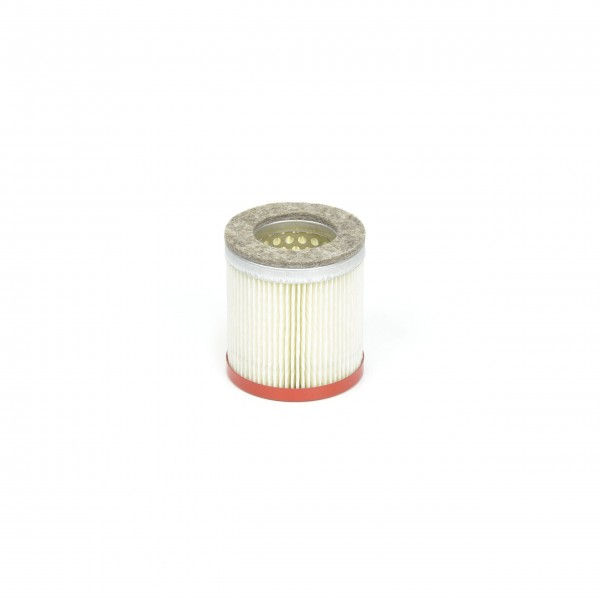# 90950500000 Filter CartridgeDelivery time: 1 - 3 business days

• 90950500000
Becker Original Filter Cartridge 90950500000 The filter medium's (paper) filtration... more
Product information "90950500000 Filter Cartridge"

Becker Original Filter Cartridge 90950500000
The filter medium's (paper) filtration efficiency is 99% at 2 µm. Filter cartridges made of paper can be cleaned by blowing through with clean dry compressed air. To ensure proper function of your Becker product, filter elements have to be cleaned and changed regularly according to the operation manual.

 Dimensions [mm]: 64-38-70 Filtration efficiency: 99% at 2 µm Use of filter cartridge: DT/T/VT 3.25/3.40, D Filtration: Filter Cartridges Filter material: paperDT 3.25 (Index D) Order number: DT3.25-DDT 3.25 (Index E) Order number: DT3.25-EDT 3.25 (Index F) Order number: DT3.25-FDT 3.25 (Index G) Order number: DT3.25-GDT 3.40 Order number: DT3.40DT 3.40 (Index A) Order number: DT3.40-ADT 3.40 (Index B) Order number: DT3.40-BDT 3.40 (Index C) Order number: DT3.40-CDT 3.40 (Index D) Order number: DT3.40-DDT 3.40 (Index E) Order number: DT3.40-EDT 3.40 (Index F) Order number: DT3.40-FDT 3.40 (Index G) Order number: DT3.40-GDT 3.40 K Order number: DT3.40KDT 3.40 K (Index A) Order number: DT3.40K-ADT 3.40 K (Index B) Order number: DT3.40K-BDT 3.40 K (Index D) Order number: DT3.40K-DDT 3.40 K (Index E) Order number: DT3.40K-EDT 3.40 K (Index F) Order number: DT3.40K-FDT 3.40 K (Index G) Order number: DT3.40K-G DT 4.25 K (Index A) Order number: DT4.25K-A DT 4.25 K (Index C) Order number: DT4.25K-CDT 4.25 K (single-phase AC) (Index A) Order number: DT4.25Ksingle-phaseAC-A DT 4.25 K/0-06 (Index A) Order number: DT4.25K0-06-ADT 4.25 K/0-06 (three-phase AC) (Index A) Order number: DT4.25K0-06three-phaseAC-A DT 4.25/0-80 (Index B) Order number: DT4.250-80-B DT 4.25/0-80 (Index C) Order number: DT4.250-80-C DT 4.40 K (Index A) Order number: DT4.40K-A DT 4.40 K (Index C) Order number: DT4.40K-C DT 4.40 K/0-400 Order number: DT4.40K0-400 DT 4.40 K/0-400 (Index A) Order number: DT4.40K0-400-A DT 4.40 K/0-400 (Index C) Order number: DT4.40K0-400-CDT 4.40 K/5-63 (three-phase AC) (Index A) Order number: DT4.40K5-63three-phaseAC-ADT 4.40 K/5-63 (three-phase AC) (Index C) Order number: DT4.40K5-63three-phaseAC-C DT 4.40/0-80 (Index A) Order number: DT4.400-80-A DT 4.40/0-80 (Index C) Order number: DT4.400-80-CDVT 2.100 Order number: DVT2.100DVT 2.100 (Index A) Order number: DVT2.100-ADVT 2.100 (Index B) Order number: DVT2.100-BDVT 2.100 (Index C) Order number: DVT2.100-CDVT 2.100 (Index D) Order number: DVT2.100-DDVT 2.140 Order number: DVT2.140DVT 2.140 (Index A) Order number: DVT2.140-ADVT 2.140 (Index B) Order number: DVT2.140-BDVT 2.140 (Index C) Order number: DVT2.140-CDVT 2.140 (Index D) Order number: DVT2.140-DDVT 2.60 Order number: DVT2.60DVT 2.80 Order number: DVT2.80DVT 2.80 (Index A) Order number: DVT2.80-ADVT 2.80 (Index B) Order number: DVT2.80-BDVT 2.80 (Index C) Order number: DVT2.80-CDVT 2.80 (Index D) Order number: DVT2.80-DDVT 2.80 (Index E) Order number: DVT2.80-ET 3.25 DSK (Index D) Order number: T3.25DSK-DT 3.25 DSK (Index E) Order number: T3.25DSK-ET 3.25 DSK (Index F) Order number: T3.25DSK-FT 3.25 DSK (Index G) Order number: T3.25DSK-GT 3.25 DSK, CSA (Index E) Order number: T3.25DSKCSA-ET 3.25 DSK, CSA (Index F) Order number: T3.25DSKCSA-FT 3.25 DSK, CSA (Index G) Order number: T3.25DSKCSA-GT 3.40 DSK Order number: T3.40DSKT 3.40 DSK (Index A) Order number: T3.40DSK-AT 3.40 DSK (Index B) Order number: T3.40DSK-BT 3.40 DSK (Index C) Order number: T3.40DSK-CT 3.40 DSK (Index D) Order number: T3.40DSK-DT 3.40 DSK (Index E) Order number: T3.40DSK-ET 3.40 DSK (Index F) Order number: T3.40DSK-FT 3.40 DSK (Index G) Order number: T3.40DSK-G T 4.25 DSK (Index A) Order number: T4.25DSK-A T 4.25 DSK (Index C) Order number: T4.25DSK-C T 4.25 DSK (Index X) Order number: T4.25DSK-X T 4.25 DSK (Index Z) Order number: T4.25DSK-Z T 4.25 DV (Index A) Order number: T4.25DV-A T 4.25 DV (Index C) Order number: T4.25DV-C T 4.25 DV (Index X) Order number: T4.25DV-X T 4.25 DV (Index Z) Order number: T4.25DV-Z T 4.40 DSK (Index A) Order number: T4.40DSK-A T 4.40 DSK (Index C) Order number: T4.40DSK-C T 4.40 DSK (Index X) Order number: T4.40DSK-X T 4.40 DSK (Index Z) Order number: T4.40DSK-ZT 4.40 DSK, /-34 (Index A) Order number: T4.40DSK-34-AT 4.40 DSK, /-34 (Index C) Order number: T4.40DSK-34-CT 4.40 DSK, /-34 (Index X) Order number: T4.40DSK-34-XT 4.40 DSK, /-34 (Index Z) Order number: T4.40DSK-34-ZT 4.40 DV (Index A) Order number: T4.40DV-A T 4.40 DV (Index C) Order number: T4.40DV-C T 4.40 DV (Index X) Order number: T4.40DV-X T 4.40 DV (Index Z) Order number: T4.40DV-ZVT 3.25 (Index D) Order number: VT3.25-DVT 3.25 (Index E) Order number: VT3.25-EVT 3.25 (Index F) Order number: VT3.25-FVT 3.25 (Index G) Order number: VT3.25-GVT 3.40 Order number: VT3.40VT 3.40 (Index A) Order number: VT3.40-AVT 3.40 (Index B) Order number: VT3.40-BVT 3.40 (Index C) Order number: VT3.40-CVT 3.40 (Index D) Order number: VT3.40-DVT 3.40 (Index E) Order number: VT3.40-EVT 3.40 (Index F) Order number: VT3.40-FVT 3.40 (Index G) Order number: VT3.40-GVT 3.60 Order number: VT3.60 VT 4.25 Order number: VT4.25 VT 4.25 (Index A) Order number: VT4.25-A VT 4.25 (Index B) Order number: VT4.25-B VT 4.25 (Index C) Order number: VT4.25-C VT 4.40 Order number: VT4.40 VT 4.40 (Index A) Order number: VT4.40-A VT 4.40 (Index C) Order number: VT4.40-C VT 4.40/0-400 Order number: VT4.400-400VT 4.40/0-400 (Index C) Order number: VT4.400-400-C VT 4.40/0-65 (Index A) Order number: VT4.400-65-A VT 4.40/0-65 (Index C) Order number: VT4.400-65-C VT 4.40/0-90 (Index B) Order number: VT4.400-90-B VT 4.40/0-90 (Index C) Order number: VT4.400-90-C
VT 4.40
Content 1 unit
VT 4.25
Content 1 unit
VT 4.40 (Index A)
Content 1 unit
Viewed